# Experiment 8 Double Replacement Reactions Background: Some reactions have the net effect of causing the cation...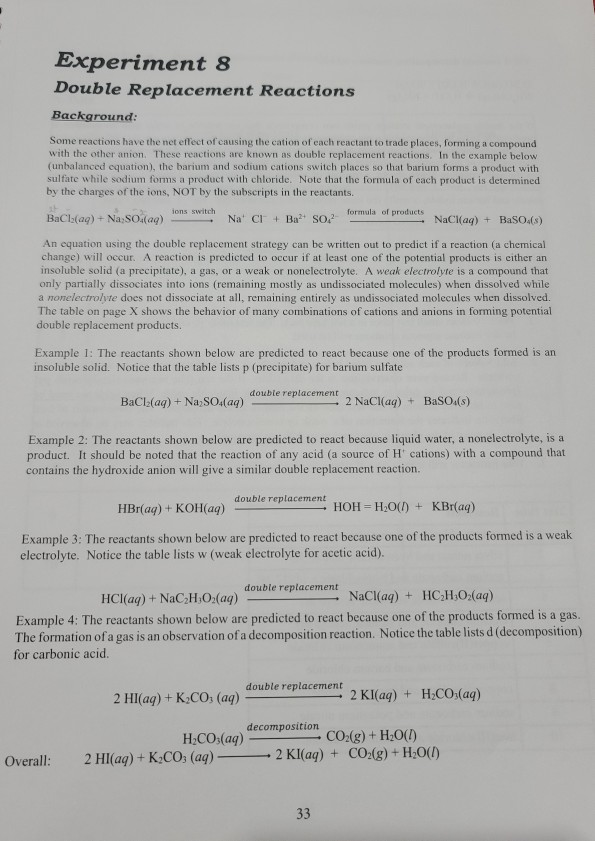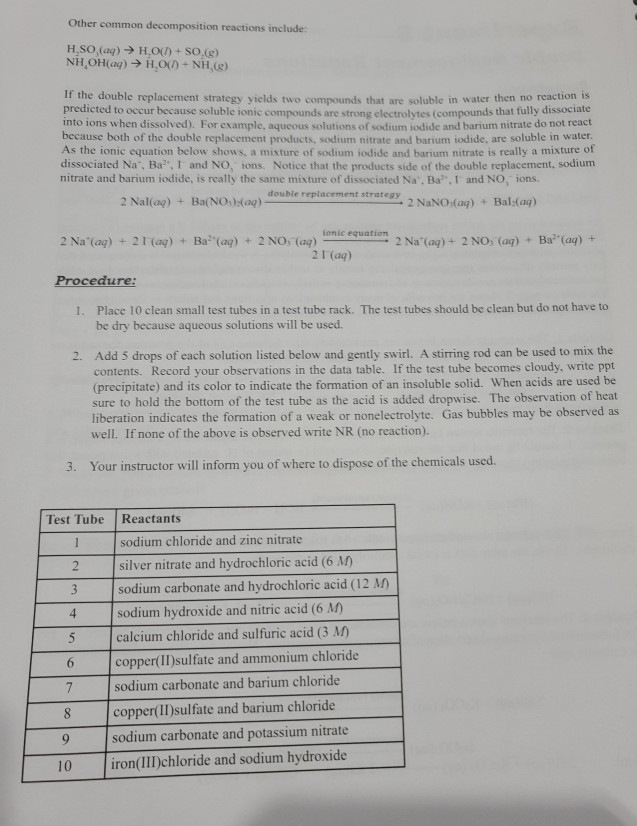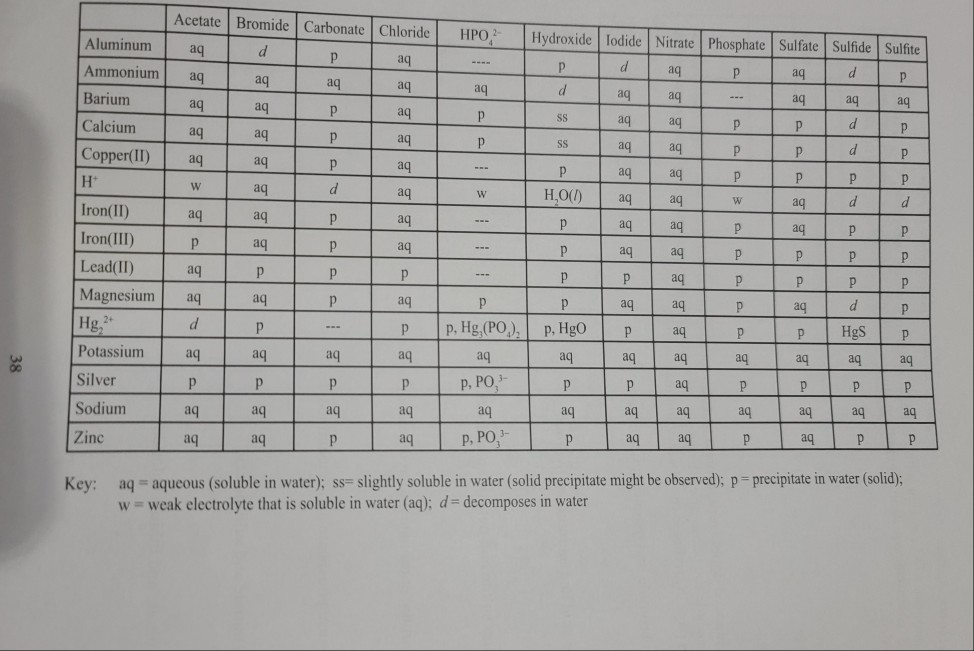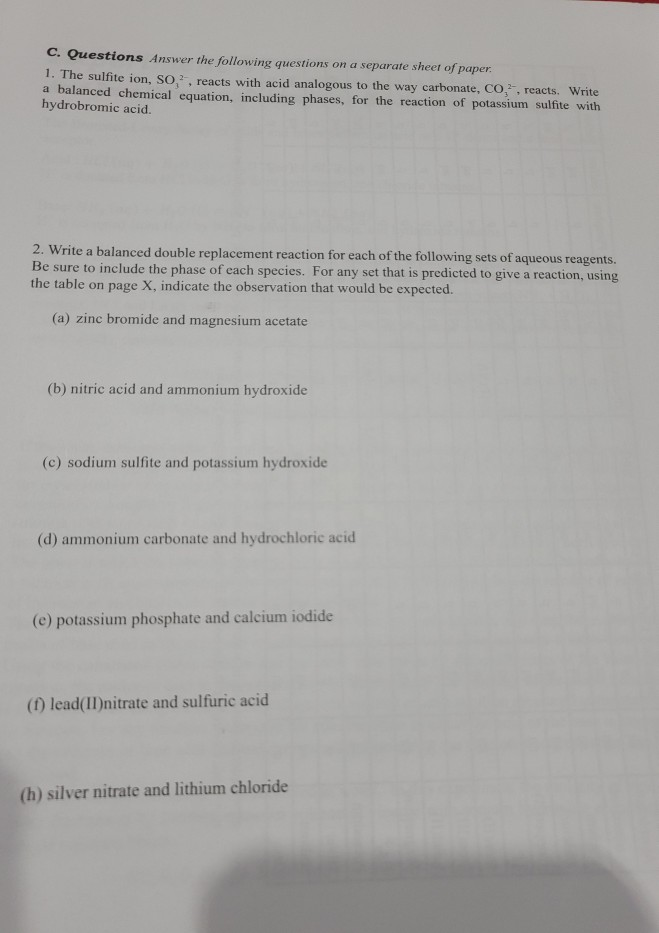Experiment 8 Double Replacement Reactions Background: Some reactions have the net effect of causing the cation of each reactant to trade places, forming a compound with the other anion. These reactions are known as double replacement reactions. In the example below (unbalanced equation), the barium and sodium cations switch places so that barium forms a product with sulfate while sodium forms a product with chloride. Note that the formula of each product is determined by the charges of the ions, NOT by the subscripts in the reactants. BaCl(aq) +NaSO4(ag) - Na Cl + BaSO? formula of products - NaClaq) + BaSO4(s) An equation using the double replacement strategy can be written out to predict if a reaction (a chemical change) will occur. A reaction is predicted to occur if at least one of the potential products is either an insoluble solid (a precipitate), a gas, or a weak or nonelectrolyte. A weak electrolyte is a compound that only partially dissociates into ions (remaining mostly as undissociated molecules) when dissolved while a nonelectrolyte does not dissociate at all, remaining entirely as undissociated molecules when dissolved. The table on page X shows the behavior of many combinations of cations and anions in forming potential double replacement products. Example 1: The reactants shown below are predicted to react because one of the products formed is an insoluble solid. Notice that the table lists p (precipitate) for barium sulfate double replacement BaCl(aq) + Na, SO4(aq) 2 NaCl(aq) + BaSO4(s) Example 2: The reactants shown below are predicted to react because liquid water, a nonelectrolyte, is a product. It should be noted that the reaction of any acid (a source of H' cations) with a compound that contains the hydroxide anion will give a similar double replacement reaction. HBr(aq) + KOH(aq) double replacement HOH = H2O(0) + KBr(aq) Example 3: The reactants shown below are predicted to react because one of the products formed is a weak electrolyte. Notice the table lists w (weak electrolyte for acetic acid). double replacement HCl(aq) + NaC2H,O,(aq) -NaCl(aq) + HCH.Oxaq) Example 4: The reactants shown below are predicted to react because one of the products formed is a gas. The formation of a gas is an observation of a decomposition reaction. Notice the table lists d (decomposition) for carbonic acid double replacement 2 Hl(aq) + K,CO, (aq) - 2 Kl(aq) + H2CO3(aq) decomposition H.CO:(aq) - CO2(g) + H2O(1) 2 Hl(aq) + K:CO, (ag) 2 Kl(aq) + CO2(g) + H2O(l) Overall:
Other common decomposition reactions include: H.SO (aq) → H.O() + SO.(g) NHOH(aq) + H2O + NH,(g) If the double replacement strategy yields two compounds that are soluble in water then no ra predicted to occur because soluble ionic compounds are strane electrolytes compounds that fully dissociad into ions when dissolved). For example, aqueous solutions of sodium iodide and barium nitrate do not because both of the double replacement products, sodium nitrate and barium iodide, are soluble in water As the ionic equation below shows, a mixture of sodium iodide and barium nitrate is really a mixture of dissociated Na Ba and NO, ons. Notice that the products side of the double replacement, sodium nitrate and barium iodide, is really the same mixture of dissociated Na. Bay. and NO, ions. double replacement strategy 2 Nal(aq) + Ba(NO)(aq) - - 2 NaNO (aq) + Bal:(04) tonic equation 2 Na'(aq) + 2 NO, (aq) + Ba(aq) + 2 Na (aq) + 2 (aq) + Ba(aq) + 2 NO, (aq) 2 (aq) Procedure: 1. Place 10 clean small test tubes in a test tube rack. The test tubes should be clean but do not have to be dry because aqueous solutions will be used. 2. Add 5 drops of each solution listed below and gently swirl. A stirring rod can be used to mix the contents. Record your observations in the data table. If the test tube becomes cloudy, write ppt (precipitate) and its color to indicate the formation of an insoluble solid. When acids are used be sure to hold the bottom of the test tube as the acid is added dropwise. The observation of heat liberation indicates the formation of a weak or nonelectrolyte. Gas bubbles may be observed as well. If none of the above is observed write NR (no reaction). 3. Your instructor will inform you of where to dispose of the chemicals used. Test Tube Reactants sodium chloride and zinc nitrate 2 silver nitrate and hydrochloric acid (6 M) 3 sodium carbonate and hydrochloric acid (12 M) 4 sodium hydroxide and nitric acid (6 M) 5 calcium chloride and sulfuric acid (3 M copper(II)sulfate and ammonium chloride sodium carbonate and barium chloride 8 copper(II)sulfate and barium chloride 9 sodium carbonate and potassium nitrate 10 iron(III)chloride and sodium hydroxide
H Acetate Bromide Carbonate Chloride HPO Hydroxide Todide Nitrate Phosphate Sulfate Sulfide Sulfite | Aluminum aq d p aq .... daqpaq dp Ammoniumaq|aqaqaqaqdaqaq aqaqaq Barium aq aqp aq P SS aq aq P P dP Calcium aq aqpaq P saqaq PPdpl Copper(II) aq aq p a q --- p aq aq р р р р w aq d aq H.0(1) I aq aq w aga d Iron(II) aq aq aq I . I P ] I aq aq aq P P Iron(III) P aqp aq aq I --- . I Plaq aq aq P aq P P P P Lead(II) I aq PPP - IP IP aq PPP Magnesium aqaq paqp paqaq paqd|p Hg, Id P I ... IP p, Hg, (PO), p. Hgo e aq e IP Hgsel Potassium aqaqaqaqaqaqaqaqaqaq aq aq р т р р | Silver т р Тр, PO, Ip p aq р р т р р Sodium aqaqaqaqaqaqaqaqaqaqaqaq Zinc e aq IPP aq p I aq aq p, PO, Ipaq 38 aq Key: aq = aqueous (soluble in water); ss=slightly soluble in water (solid precipitate might be observed); p = precipitate in water (solid); w weak electrolyte that is soluble in water (aq); d = decomposes in water
c. Questions Answer the following questions on a separate sheet of paper. 1. The sulfite ion, SO, reacts with acid analogous to the way carbonate, CO,.reacts. Write a balanced chemical equation, including phases, for the reaction of potassium sulfite with hydrobromic acid. 2. Write a balanced double replacement reaction for each of the following sets of aqueous reagents. Be sure to include the phase of each species. For any set that is predicted to give a reaction, using the table on page X, indicate the observation that would be expected. (a) zinc bromide and magnesium acetate (b) nitric acid and ammonium hydroxide (c) sodium sulfite and potassium hydroxide (d) ammonium carbonate and hydrochloric acid (e) potassium phosphate and calcium iodide (1) lead(II) nitrate and sulfuric acid (h) silver nitrate and lithium chloride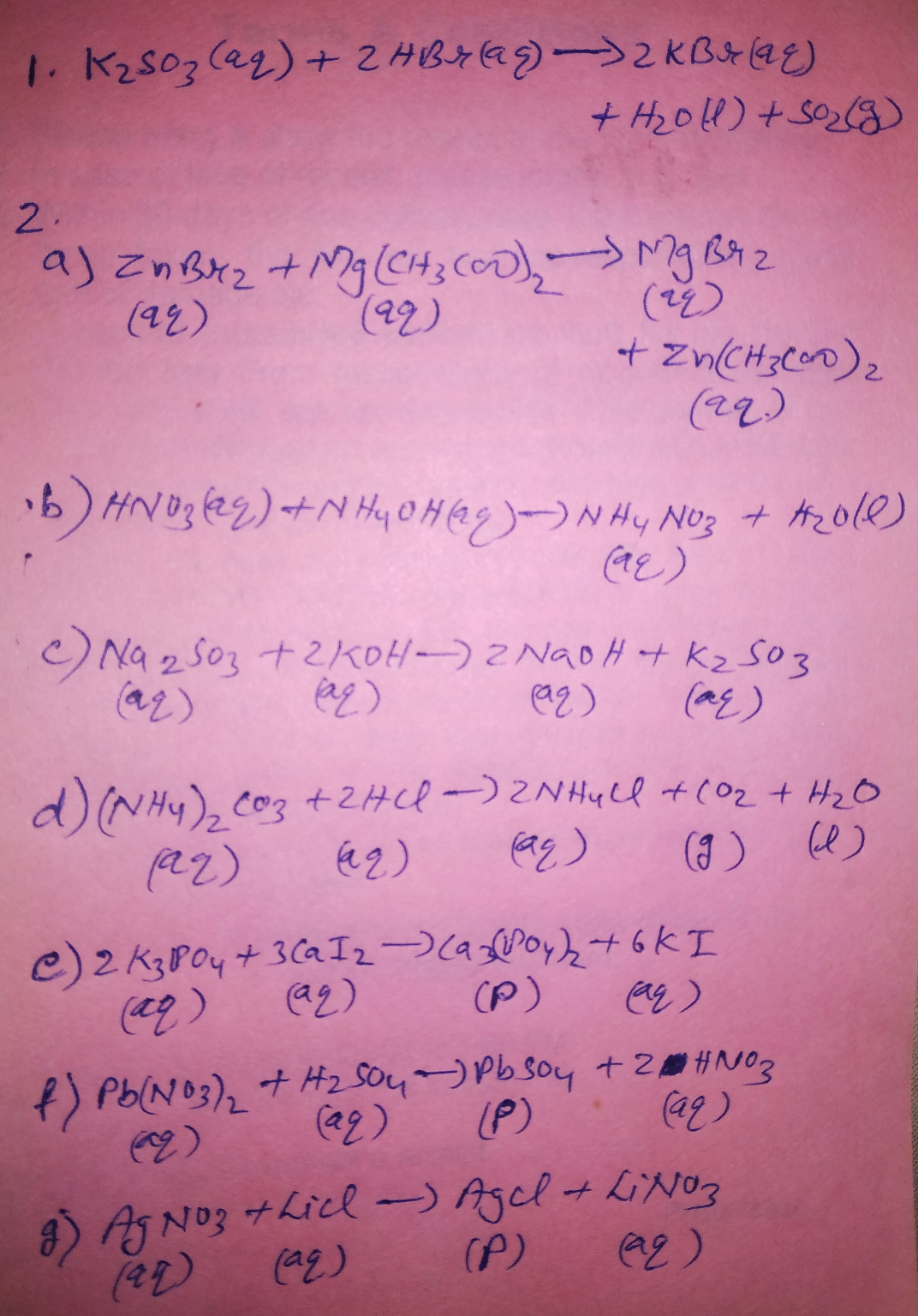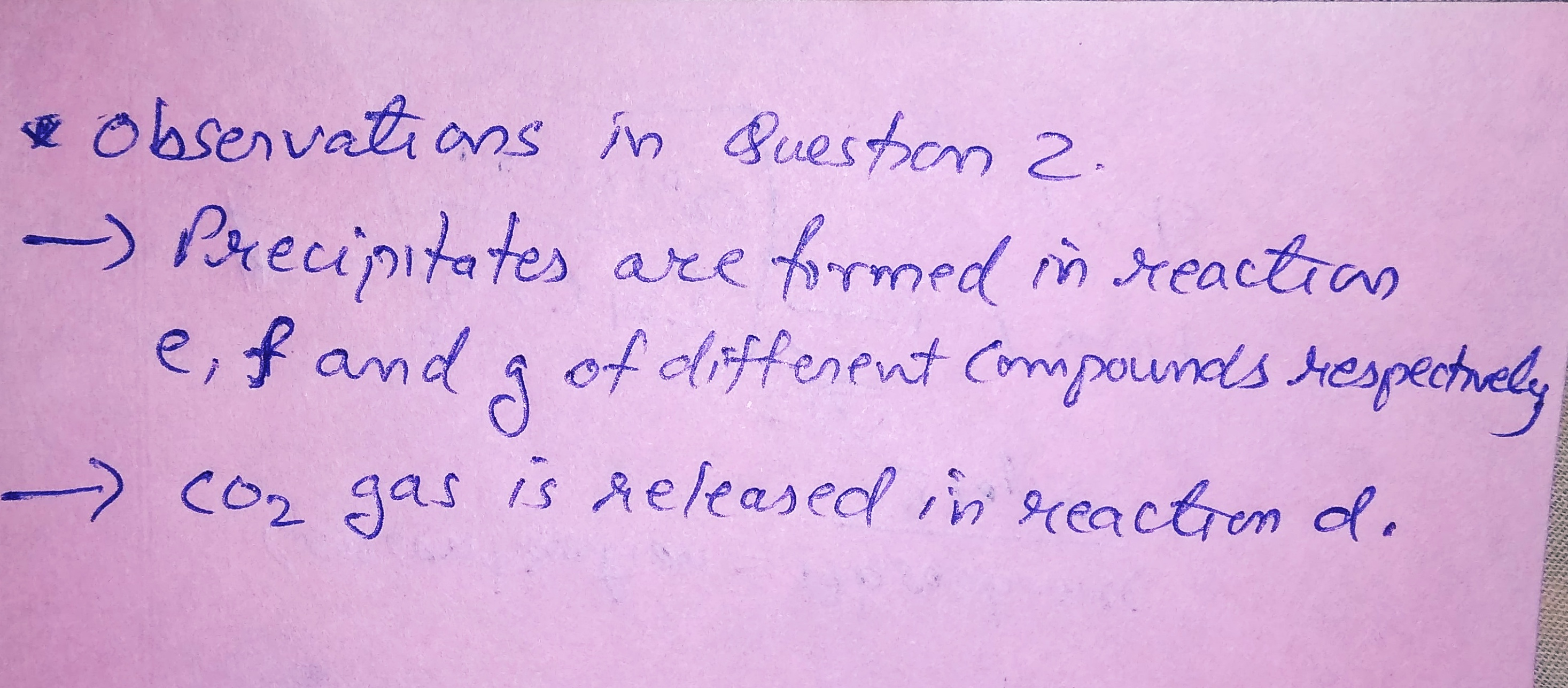#### Earn Coin

Coins can be redeemed for fabulous gifts.

Similar Homework Help Questions
• ### When you write the answers, please write the page number for which the question is located....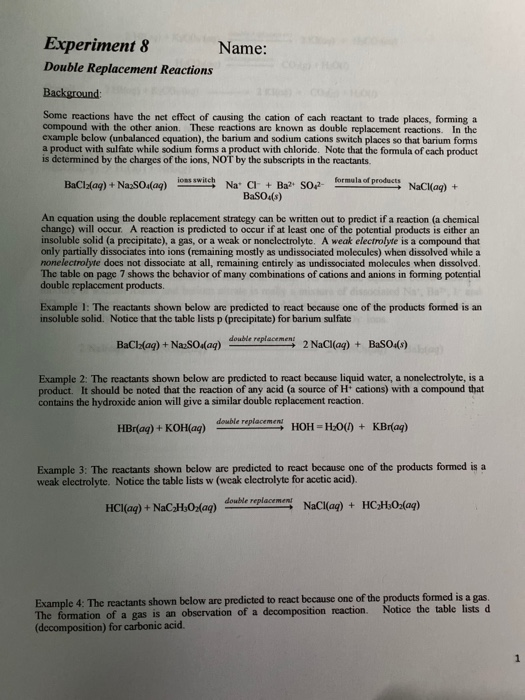When you write the answers, please write the page number for which the question is located. Thank you! Experiment 8 Name: Double Replacement Reactions Background: Some reactions have the net effect of causing the cation of cach reactant to trade places, forming a compound with the other anion. These reactions are known as double replacement reactions. In the example below (unbalanced equation), the barium and sodium cations switch places so that barium forms a product with sulfate while sodium forms...

• ### Practice Problem 7. Write a balanced chemical reaction for each of the following double replacement reactions....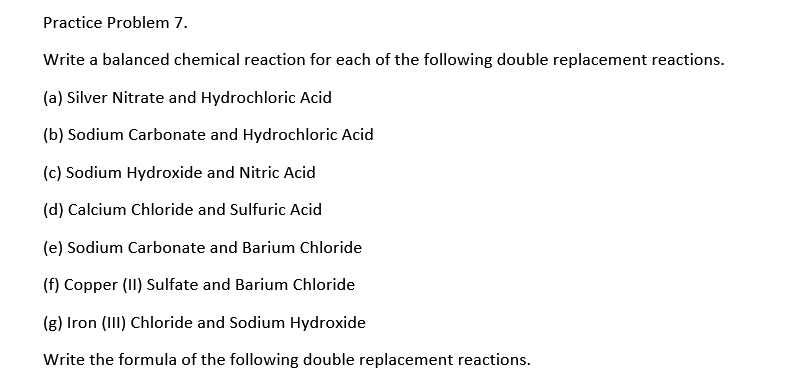Practice Problem 7. Write a balanced chemical reaction for each of the following double replacement reactions. (a) Silver Nitrate and Hydrochloric Acid (b) Sodium Carbonate and Hydrochloric Acid (c) Sodium Hydroxide and Nitric Acid (d) Calcium Chloride and Sulfuric Acid (e) Sodium Carbonate and Barium Chloride (f) Copper (II) Sulfate and Barium Chloride (8) Iron (III) Chloride and Sodium Hydroxide Write the formula of the following double replacement reactions.

• ### FOR OUESTIONS 25- 34. For all single replacement reactions below predict whether or not the reaction...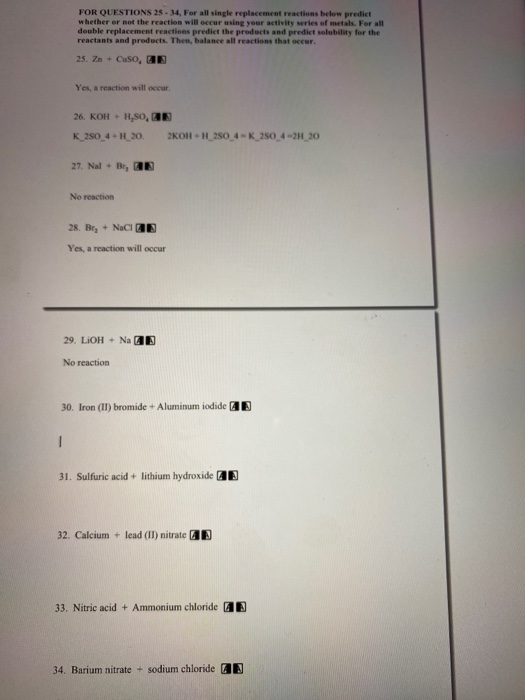FOR OUESTIONS 25- 34. For all single replacement reactions below predict whether or not the reaction will occur using your activity series of metals. For all double replacement reactions predict the products and predict solubility for the reactants and products. Then, balance all reactions that occur. 25. Zn + Cuso, AB Yes, a reaction will occur. 26. KOH + H,SO, K_250_4+H_20. 2KOH + H_250_4-K_250_4-2H_20 27. Nal + B, AB No reaction 28. Bry + NaCl AB Yes, a reaction will...

• ### Double Replacement potassium carbonate (aq) + silver nitrate (aq) → potassium nitrate (aq) + silver carbonate...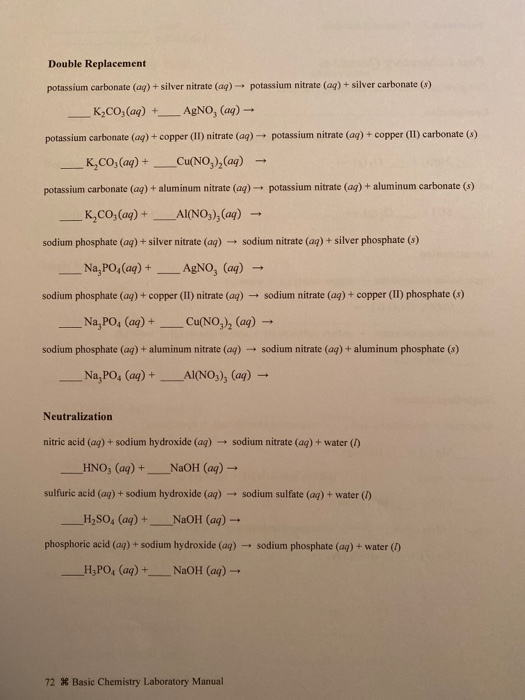Double Replacement potassium carbonate (aq) + silver nitrate (aq) → potassium nitrate (aq) + silver carbonate (s) K2CO3(aq) + AgNO, (ag) - potassium carbonate (aq) + copper (II) nitrate (aq) potassium nitrate (aq) + copper (II) carbonate (8) K,CO, (aq) + _Cu(NO, ),(aq) potassium carbonate (aq) + aluminum nitrate (aq) + potassium nitrate (aq) + aluminum carbonate (3) K.CO; (aq) + _Al(NO3) (aq) - sodium phosphate (aq) + silver nitrate (aq) sodium nitrate (aq) + silver phosphate (8) Na, PO...

• ### Write a balanced equation for the double-replacement precipitation reaction described, using the smallest possible integ...

Write a balanced equation for the double-replacement precipitation reaction described, using the smallest possible integer coefficients. 1. A precipitate forms when aqueous solutions of lead(II) nitrate and barium chloride are combined. 2. A precipitate forms when aqueous solutions of iron(III) sulfate and barium hydroxide are combined. 3. A reaction occurs when aqueous solutions of sodium hydroxide and hydrochloric acid are combined. 4. A reaction occurs when aqueous solutions of potassium hydroxide and hydrosulfuric acid are combined. Assume excess base.

• ### Identify each of the following reactions as synthesis, decomposition, combustion, single replacement, or double replacement. If...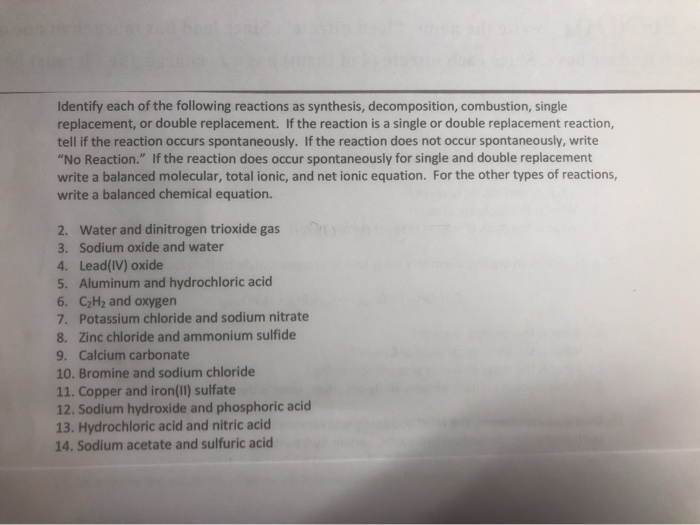Identify each of the following reactions as synthesis, decomposition, combustion, single replacement, or double replacement. If the reaction is a single or double replacement reaction, tell if the reaction occurs spontaneously. If the reaction does not occur spontaneously, write "No Reaction." If the reaction does occur spontaneously for single and double replacement write a balanced molecular, total ionic, and net ionic equation. For the other types of reactions, write a balanced chemical equation. 2. Water and dinitrogen trioxide gas 3....

• ### Only do 31,32,33,34 please!!! do not need the others FOR QUESTIONS 25-34For all single replacement reactions...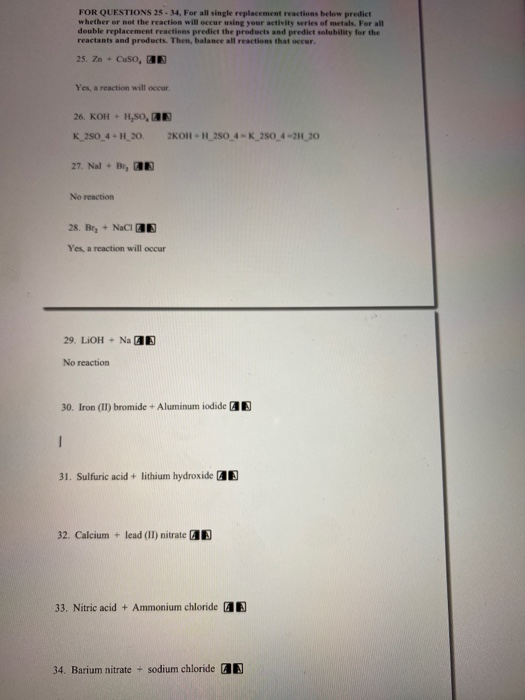Only do 31,32,33,34 please!!! do not need the others FOR QUESTIONS 25-34For all single replacement reactions below predict whether or not the reaction will occur using your activity series of metals. For all double replacement reactions predict the products and predict solubility for the reactants and products. Then, balance all reactions that occur. 25. Zn + Cuso, AB Yes, a reaction will occur. 26. KOH + H,SO, K_250_4+H_20. 2KOH H_250_4-K_250_4-2H_20 27. Nal + B, AB No reaction 28. Bry +...

• ### 0.1M barium chloride and 3 M sulfuric acid 5. Reactants Molecular Equation Net lonic Equation Pre...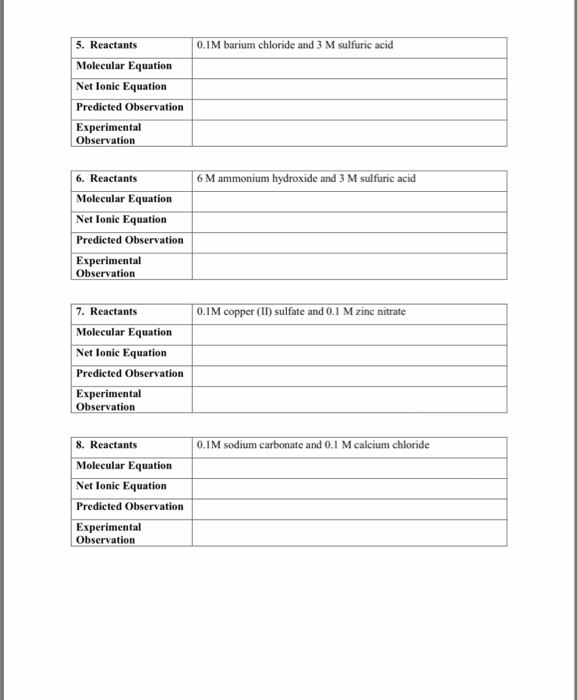0.1M barium chloride and 3 M sulfuric acid 5. Reactants Molecular Equation Net lonic Equation Predicted Observation Experimental Observation 6. Reactants Molecular Equation Net Ionic Equation Predicted Observation Experimental 6 M ammonium hydroxide and 3 M sulfuric acid Observation 7. Reactants Molecular Equation Net lonic Equation Predicted Observation Experimental 0.1M copper (II) sulfate and 0.1 M zinc nitrate Observation 8. Reactants Molecular Equation Net lonic Equation Predicted Observation Experimental 0.1M sodium carbonate and 0.1 M calcium chloride Observation 9. Reactants...

• ### copper(II) sulfate + zine nitrate (Cuso+Zn(NO Double Replacement Reactions Total lonic Format Net lonic Format Molecular...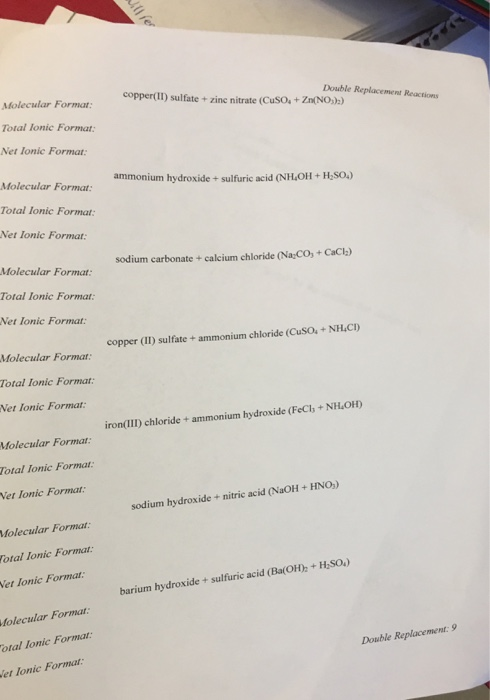copper(II) sulfate + zine nitrate (Cuso+Zn(NO Double Replacement Reactions Total lonic Format Net lonic Format Molecular Format Total lonic Format Net Ionic Format ammonium hydroxide + sulfuric acid (NH OH+HSO sodium carbonate + calcium chloride (Na CO,+ CaCl Molecular Format: Total Ionic Format: Net Ionic Format.: copper (II) sulfate+ ammonium chloride (CusO+NH CD Molecular Formar: Total Ionic Format: Net Ionic Format iron(III) chloride + ammonium hydroxide (FeCI; + NİLOH) Molecular Format: Total Ionic Format: Vet lonic Format: sodium hydroxide +...

• ### Chemical Reactions For each reaction you observed, the names of the reactants and products are given...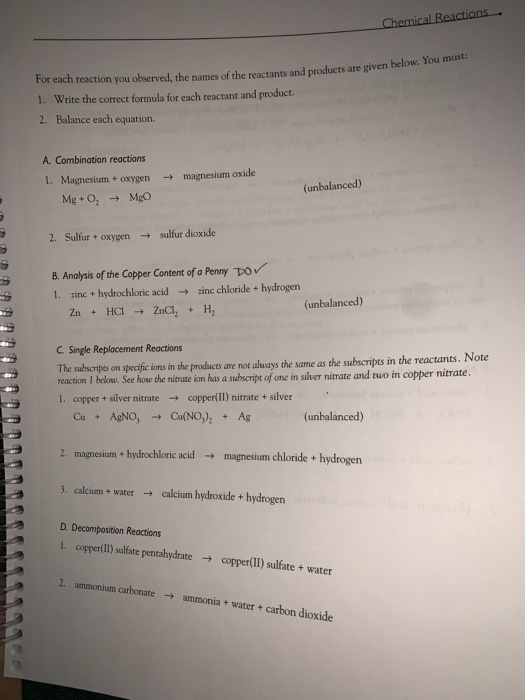Chemical Reactions For each reaction you observed, the names of the reactants and products are given below. You must: 1. Write the correct formula for each reactant and product. Balance each equation. 2. A. Combination reactions magnesium oxide 1. Magnesium + oxygen (unbalanced) Mg+ O, MgO sulfur dioxide 2. Sulfur + oxygen B. Analysis of the Copper Content of a Penny po zinc chloride + hydrogen zinc + hydrochloric acid HCI ZnCl, 1. (unbalanced) н, Zn + C. Single Replacement...